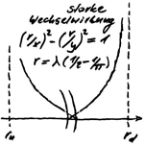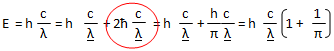# 1 THEORY OF OSCIS - Die eine Quantentheorie

Die eine Quantentheorie
Menue in German and English - select!
Es geht um die einfachste Stringtheorie nach Art der ART,
die den Aufbau der Elementarteilchen erklärt.
Phänomene und Paradoxa lassen sich math. begründen.
Benutzt werden Falsifikation und das Noether-Theorem
mit der letzten Invarianz der Quanten.

It's about the simplest string theory in the way of the GR,
which
explains the structure of the elementary particles.
Phenomena and paradoxes can be justified mathematically.
Used are falsification and the Noether theorem with
the last invariance of the quanta.
The one quantum theory
Direkt zum SeiteninhaltDiese Seite ist
frei von Esoterik
und Quantenlogik!
Die Theoie
der Oszis

## 1 THEORY OF OSCIS

ENGLISH ▶
The "theory of oszis" - TO for short - results from the falsification of light and MATTER QUANTUM HYPOTHESIS with the DILEMMA OF QT. From the point of view of the TO, Einstein's light quantum hypothesis is reinterpreted in the end - see SPOOKY PHOTON. In order to exclude disturbing influences, the development of the theory takes place in a quantum vacuum. It is therefore assumed that only those quanta are present in space that are the subject of the investigation. In order not to fall into the trap of the locality immediately, its size goes mathematically towards infinity, which does not mean that it is infinite. This is what is meant when we talk about the empty universe.
THE DILEMMA OF QUANTUM THEORY
The Quant is the very last. The attempt to approach the quantum via its properties cannot work because they are unknown. Objects with properties have the naughtiness to influence each other. If there are many, then in the end everything depends on everything. With the reduction of the objects the mutual influence can be seen through sometime. But to get to the quant, you have to go beyond it, otherwise it wouldn't be the very last thing. But this can only mean that the mutual influence must come to an end. It comes to the following statement:

A quant is only a quant if it does not make itself dependent in any of its properties on another quant!

The main idea for the solution of the problem "quantum theory" is the statement in the rank of a lemma. Due to its destructive character it is best suited for falsification! It becomes the dilemma of quantum theory - nomen est omen. The dilemma of QT stands for the last invariance in quantum theory. The corresponding association is the Noether theorem, which finally leads to the solution, i.e. to the elementary particles. The dilemma of QT replaces the "Copenhagen interpretation", i.e. its principles or adequate statements. Without them, quantum logic can be dispensed with. What quantum theory can be is no longer restricted from the outset. That this limitation exists in classical quantum theory has been established for some time (Peter Mittelstaedt, physicist and philosopher 1929-2014).
last modification 24.02.2019
THE MATTER QUANTUM HYPOTHESISWith the equation I have added the missing matter quantum hypothesis to Einstein's light quantum hypothesis. The invariance of the photon in relation to translation and rotation makes this possible. However, the law of induction makes the single circular wave impossible: Bending a circular wave as a thought experiment. A second wave had to be created, which was different from the first one. It was therefore obvious to combine Planck's constant and the reduced constant as basic quantum theoretical elements. In order to give the uncertainty relation a real background, it should definitely become real with the equation (bordered in red). At that time I was still a school boy.The first term of the above equation was interpreted as a circular wave and the second term as a dipole wave. Due to the quantum numbers of proton and neutron, the circular wave was placed in the plane of the E-field. The dipole wave should fold in the plane of the B-field, because so their charge and spin are 0. Nested into each other, the shell model of the proton and neutron resulted, analogous to the known structural equations. I succeeded in arranging them spatially in such a way that charge and spin were correct.

Only with the proof of the Higgs particle the topic was taken up again. The structure of circle wave and dipole wave got the name osci - from oscillator. Analogous to the up and down quark there is an up- and down-osci. They are primitive oscillators: The circular wave goes around once and the dipole wave is folded once. The theory that deals with them becomes the theory of the oscis - TO for short. The matter quantum hypothesis became the energy equation of the osci, and based on the reduced quantum of action λ is the reduced wavelength. Despite its name, it is of course longer than the Copmton wavelength λ:
λ = λ (1+1/π)

Only with the proof of the Higgs particle the topic was taken up again. The structure of circle wave and dipole wave got the name osci - from oscillator. Analogous to the up and down quark there is an up- and down-osci. They are primitive oscillators: The circular wave goes around once and the dipole wave is folded once. The theory that deals with them becomes the theory of the oscis - TO for short. The matter quantum hypothesis became the energy equation of the osci, and based on the reduced quantum of action λ is the reduced wavelength.

String theoretical interpretation of the energy equation of the osci: Its geometrical interpretation as an osci is only justified if the circular and dipole waves are decoupled. Decoupled thus stands for the energetic equilibrium state, which expresses the energy equation of the osci. This is what is meant by the prediction of the TO. It is therefore about the geometric interpretation of the energy equation of the osci, as a string model. With it the decoupling of the waves becomes a question of symmetry. All other geometric properties must be selected so that the waves are decoupled. In all other cases there must be a contradiction to the dilemma of TO (uniqueness by falsification). The first consequence of this is the field orientation in primitive osci:

The circular wave must be in the plane of the E-field and the dipole wave must fold in the plane of the B-field.

In addition to the electromagnetic interaction, there is also a strong and gravitative interaction. Only they give the osci-gyro its stability. One thing is already obvious:

The weak interaction can be omitted because the dipole wave assumes its role. And there were only three ...
last modification 28.04.2019
go to side  1  2  3  4  5  6  7  8  9
Zurück zum Seiteninhalt